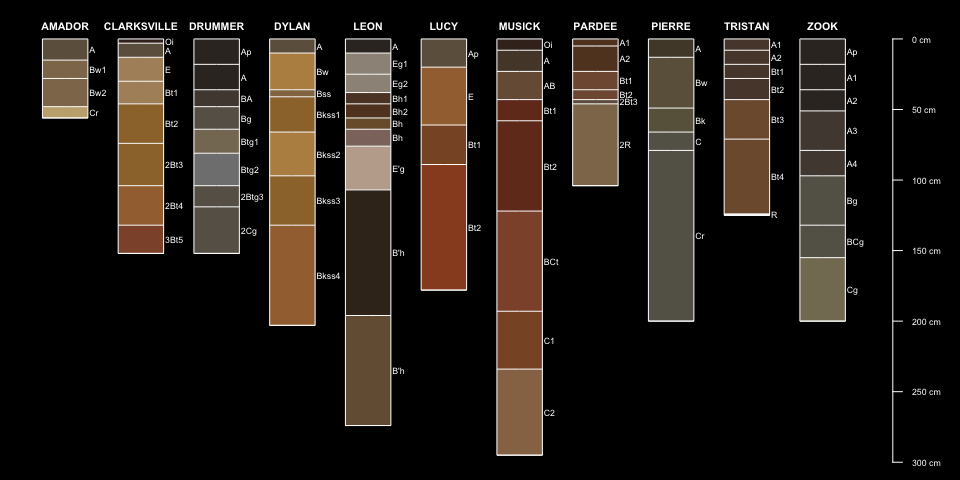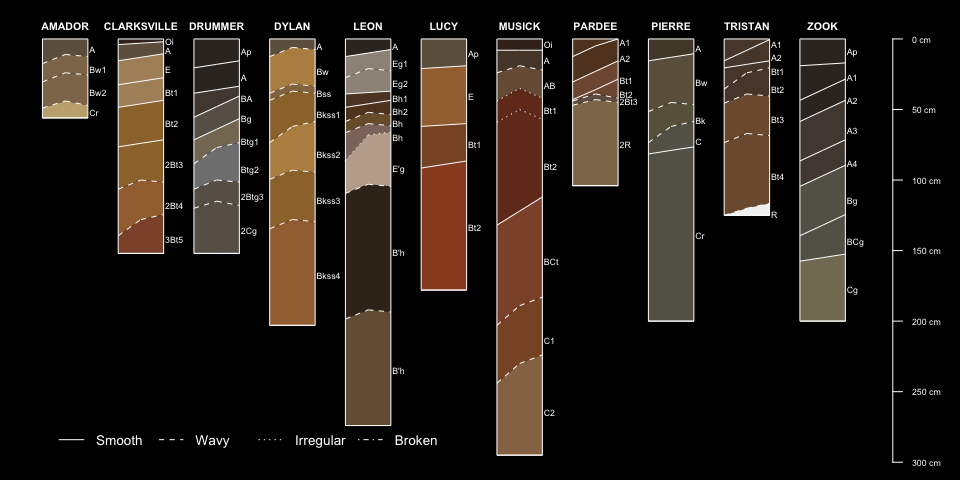1 Introduction

1.2 Visual Encoding Ideas

1.2.1 Distinctness

• diagnoal lines depict the average depth over which the boundary is defined

• do we need to use a more rigorous geometry model?
• may be hard to interpret without detailed explanation / examples
• visually compact
• simple geometry
• color blending or shaded regions of overlap

• horizon boundary line thickness

1.3 Required Packages

# stable version + dependencies
install.packages(c('aqp', 'soilDB'), dependencies = TRUE)

# development version

1.4 Setup

# required libraries
library(aqp)
library(soilDB)

1.5 Offset Encoding

hzDistinctnessCodeToOffset('G')
##  7.5

1.6 Get Some Data

# select soil series names
soil.series <- c('leon', 'musick', 'clarksville', 'pardee', 'amador', 'lucy', 'dylan', 'tristan', 'pierre', 'drummer', 'zook')

# get the data
osds <- fetchOSD(soil.series)

# encode horizon boundarydistinctness via vertical offset
osds\$hd <- hzDistinctnessCodeToOffset(
osds\$distinctness,
codes=c('very abrupt', 'abrupt', 'clear', 'gradual', 'diffuse')
)

# encode horizon boundary topography via vertical offset
osds\$hzto <- hzTopographyCodeToOffset(
osds\$topography,
codes = c('smooth', 'wavy', 'irregular', 'broken')
)

# also encode horizon boundary topography las line type
osds\$hzto.lty <- hzTopographyCodeToLineType(
osds\$topography,
codes = c('smooth', 'wavy', 'irregular', 'broken')
)

# label data source, used later
site(osds)\$source <- 'OSD'

# concise representation of hz bnd distinctness and topography
# similar to field notes
osds\$bnd.code <- sprintf(
"%s%s",
substr(osds\$distinctness, 1, 1),
substr(osds\$topography, 1, 1)
)

# remove NA
osds\$bnd.code <- gsub('NANA', '', osds\$bnd.code)

2 Horizon Sketches in {aqp}

A typical set of sketches, derived from the OSDs, is presented below. Note that horizon boundaries are depicted as horizontal lines—suggesting very abrupt/smooth (VS) horizon boundaries. We all know that VS horizon boundaries are not that common; can we do better? Examples used in this article have been sourced via fetchOSD() of the {soilDB} R package.

par(mar = c(0, 0, 0, 1), bg = 'black', fg = 'white')

plotSPC(osds, width = 0.3, cex.id = 0.66, cex.names = 0.55)Using the horizon boundary data from the OSDs and encoding via “diagonals” (distinctness) and “chevrons” (topography), we see the following. Note that horizon boundary topography has also been encoded via line type for clarity. What do you think? Is this an improvement or just clutter? Feel free to contact me with questions, suggestions, or a completely different approach to increasing the information density of these sketches. The code used to generate these figures is posted on the {aqp} GitHub site.

par(mar = c(0, 0, 0, 1), bg = 'black', fg = 'white')

plotSPC(osds, width = 0.3, hz.distinctness.offset = 'hd', hz.topography.offset = 'hzto', cex.id = 0.66, cex.names = 0.55, name = 'name', hz.boundary.lty = 'hzto.lty')

legend('bottomleft', horiz = TRUE, legend = c('Smooth', 'Wavy', 'Irregular', 'Broken'), lty = 1:4, inset = 0.05, bty = 'n', cex = 0.85)3 Encoding Horizon Distinctness and Topography

Horizon boundary distinctness describes the vertical distance over which one horizon transitions to the next. Horizon boundary topography describes the complexity of the horizon boundary. In {aqp} these two features are encoded using diagonal lines (distinctness) and “chevrons” (topography). The following figure demonstrates several possible combinations of horizon boundary distinctness and topography. Horizon depths are marked with small green symbols. The magnitude of these effects can be controlled when converting text boundary codes (e.g. CW) into representative “offsets”. The default values have been selected based on an interpretation of our Field Book for Describing and Sampling Soils. The {aqp} manual contains a detailed explanation of usage and assumptions.

# a single series
x <- filter(osds, id == 'DRUMMER')

# # full set of boundary topography codes
# g <- expand.grid(
#   distinctness = c('V', 'A', 'C', 'G', 'D'),
#   topography = c('S', 'W', 'I', 'B')
# )

# simplified set, for this example
g <- expand.grid(
distinctness = c('A', 'C', 'G', 'D'),
topography = c('S', 'W', 'I')
)

# storage for intermediate results
l <- list()
# copies of the original OSD
s <- duplicate(x, times = nrow(g))

# iterate over all possible combinations
# creating / modifying a copy of the OSD at each iteration
for(i in 1:nrow(g)) {
# current profile
ss <- s[i, ]
# set for all horizons
horizons(ss)\$hd <- hzDistinctnessCodeToOffset(g\$distinctness[i])
horizons(ss)\$ht <- hzTopographyCodeToOffset(g\$topography[i])
horizons(ss)\$ht.lty <- hzTopographyCodeToLineType(g\$topography[i])
# save modified copy
l[[i]] <- ss
}

# combine list elements -> SPC
s <- combine(l)

# associated horizon boundary codes
s\$source <- sprintf("%s%s", g\$distinctness, g\$topography)

# make a figure
par(mar=c(0,0,2,1), bg = 'black', fg = 'white')
plotSPC(s, width=0.33, print.id=TRUE, hz.distinctness.offset = 'hd', hz.topography.offset = 'ht', label='source', cex.names=0.8, name = NA, color = NA, default.color = 'black', hz.boundary.lty = 'ht.lty')
title('Horizon Boundary Types', line = 0, col.main = 'white')

# label original horizon depths
p.seq <- rep(1:length(s), each = nrow(s[1, ]))
points(x = p.seq, y = s\$bottom, pch = 15, col = 'green', cex = 0.5)

# line type legend
legend('bottom', horiz = TRUE, legend = c('Smooth', 'Wavy', 'Irregular', 'Broken'), lty = 1:4, inset = 0.1)